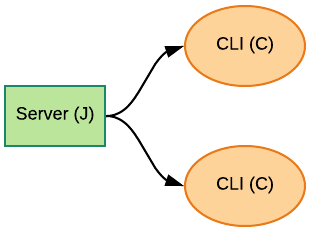## Preliminary Setup

In this quick start, we use the Docker-based JAMScript installation because it is quite straightforward to get going. Follow the instructions in the Docker installation page to get the tools and images for the Docker-based JAMScript.

## A Calculator Example

We will use a simple calculator example in this quick start. The calculator is implemented in two parts: server and client. The server is responsible for actually performing the calculations and the client is responsible for implementing the command line interface (CLI) to perform the I/O. The server that performs the calculations is implemented as a J node while the C node implements the CLI. We can run multiple CLI (C) nodes with a single calculating server as shown in the figure below.The listing below shows the JAMScript enhanced C code that implements the C node. In this particular example there is no JAMScript specific constructs in the C code. You will notice that the four functions (`add`, `subtract`, `multiply`, `divide`) used for computations are not implemented in the C side. They are implemented in the J (JavaScript) side.

``````#include <stdio.h>

// Prototypes for the functions exported from the J side
int subtract(int, int);
int multiply(int, int);
int divide(int, int);

int main() {

char operator;
int num1, num2;

while(1) {
printf("Enter an operator (+, -, *, /) or q to quit:");
scanf("%c", &operator);
if(operator == 'q' ) {
exit(0);
}
printf("Enter the first integer operand: ");
scanf("%i", &num1);

printf("Enter the second integer operand: ");
scanf("%i", &num2);

switch(operator) {
case '+':
printf("%i + %i = %i\n", num1, num2, add(num1, num2));
break;
case '-':
printf("%i - %i = %i\n", num1, num2, subtract(num1, num2));
break;
case '*':
printf("%i * %i = %i\n", num1, num2, multiply(num1, num2));
break;
case '/':
printf("%i / %i = %i\n", num1, num2, divide(num1, num2));
break;
// operator doesn't match any case constant (+, -, *, /)
default:
printf("Error! operator is not correct\n");
}

//Lazy input clear
int c;
while ((c = getchar()) != '\n' && c != EOF) {}
}
return 0;
}
``````

Now, lets looks at the J side. The J side introduces a JAMScript keyword - jsync. Simply by putting this keyword in front of a function we have asked the compiler to export the function to the C side. Of course, proper matching C prototypes in the C side (as shown in the above code block) are necessary to complete the export.

``````jsync function add(num1, num2) {
return num1 + num2;
}

jsync function subtract(num1, num2) {
return num1 - num2;
}

jsync function multiply(num1, num2) {
return num1 * num2;
}

jsync function divide(num1, num2) {
return num1 / num2;
}
``````

Save the C code block as `calc.c` and J code block as `calc.js`. Now, run the following command to compile the files.

``````djam pull mahes25/jamscript
djam compile calc.c calc.js
``````

After running the above commands, you should see the JAMScript executable `calc.jxe` in the directory where the source files are located. The `djam pull` command brought in a docker image with JAMScript language. The `djam compile` command compiled the source files inside a docker container. The resulting executable `calc.jxe` is copied back to the original location.

To run `calc.jxe`, issue the following commands.

``````djam init ideal
djam run calc.jxe --num=2 --bg
``````

The `djam init` command is setting up the system configuration for the sole Docker container. The `djam run` command runs the `calc.jxe` program in the Docker container. The command runs a single J node and two C nodes (observe the –num option).

Now, run `jam list` to see the status of the execution.

You should see a listing like the following.

``````ID         NAME      PROGRAM         HOST         D-STORE       TYPE C-NODES    TMUX-ID
app-457        app-n        calc 76b9053e2844     docker:6379     device       2 u-501-device-461-dev
``````

Since we did not specify the application name the `djam run` with the –app option, the default application (app-n) is used in the execution. Remember the command line interface (CLI) is running in the C node. So, to interact with the application we need to open a terminal to the C node. Here, we have two C nodes running. The tmux id for the J node is shown in the above listing. You can append `-` and node id of the C node to get the tmux id for the C node.

Run the following commands to see the two terminals.

``````jam term u-501-device-461-dev-1
jam term u-501-device-461-dev-2
``````

We are using tmux. So, to detach press `Ctrl-B d`.

To stop the application executing in the above listing, we need to run the following command.

``````jam kill app-457
``````